# Chapter 9 Circles NCERT Exemplar Solutions Exercise 9.1 Class 10 Maths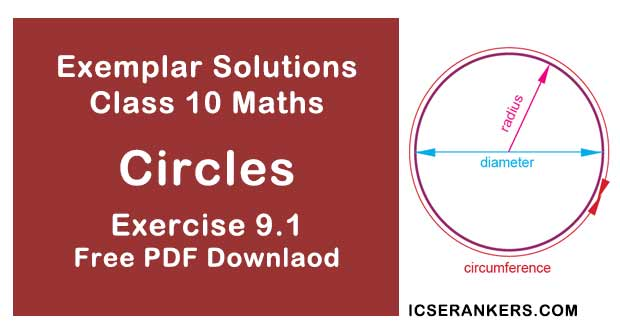Chapter Name NCERT Maths Exemplar Solutions for Chapter 9 Circles Exercise 9.1 Book Name NCERT Exemplar for Class 10 Maths Other Exercises Exercise 9.2Exercise 9.3Exercise 9.4 Related Study NCERT Solutions for Class 10 Maths

### Exercise 9.1 Solutions

Multiple Choice Questions

Choose the correct answer from the given four options :

1. 1. If radii of two concentric circles are 4 cm and 5 cm, then the length of each chord of one circle which is tangent to the other circle is
(A) 3 cm
(B) 6 cm
(C) 9 cm
(D) 1 cm

Solution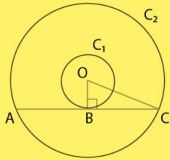As given in the question,
OA = 4cm,
OB = 5cm
And,
OA ⊥ BC
Therefore,
OB2 = OA2 + AB2
⇒ 52 = 42 + AB2
⇒ AB = 3cm
And,
BC = 2AB
= 2 × 3cm
= 6cm

2. In Fig., if AOB = 125°, then COD is equal to
(A) 62.5°
(B) 45°
(C) 35°
(D) 55°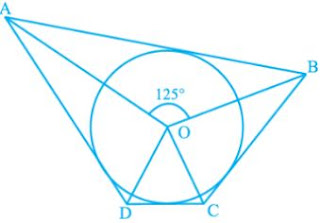Solution
ABCD is a quadrilateral circumscribing the circle
And we know that the opposite sides of a quadrilateral circumscribing a circle subtend
supplementary angles at the center of the circle.
So,
∠AOB + ∠COD = 180°
⇒ 125° + ∠COD = 180°
⇒ ∠COD = 55°

3. In Fig., AB is a chord of the circle and AOC is its diameter such that ∠ACB = 50°. If AT is the tangent to the circle at the point A, then ∠BAT is equal to
(A) 65°
(B) 60°
(C) 50°
(D) 40°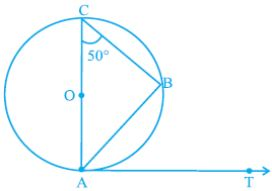Solution
As given in the question,
A circle with centre O, diameter AC and
∠ACB = 50°
AT is a tangent to the circle at point A
As, angle in a semicircle is a right angle
∠CBA = 90°
So using angle sum property of a triangle,
∠ACB + ∠CAB + ∠CBA = 180°
⇒ 50° + ∠CAB + 90° = 180°
⇒ ∠CAB = 40°  ...(1)
As, tangent to at any point on the circle is perpendicular to the radius through point of contact,
We get,
OA ⏊ AT
⇒ ∠OAT = 90°
⇒ ∠OAT + ∠BAT = 90°
⇒ ∠CAT + ∠BAT = 90°
⇒ 40° + ∠BAT = 90° [from equation (1)]
⇒ ∠BAT = 50°

4. From a point P which is at a distance of 13 cm from the centre O of a circle of radius 5 cm, the pair of tangents PQ and PR to the circle are drawn. Then the area of the quadrilateral PQOR is
(A) 60 cm2
(B) 65 cm2
(C) 30 cm2
(D) 32.5 cm2
Solution
Construction: Draw a circle of radius 5 cm with center O.
Let P be a point at a distance of 13 cm from O.
Draw a pair of tangents, PQ and PR.
OQ = OR = radius = 5cm  ...equation (1)
And OP = 13 cm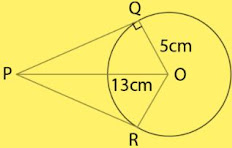Also, tangent to at any point on the circle is perpendicular to the radius through point of contact,
We get,
OQ ⏊ PQ and OR ⏊PR
△POQ and △POR are right-angled triangles.
By using Pythagoras Theorem in △PQO,
(Base)2 + (Perpendicular)2 = (Hypotenuse)2
⇒ (PQ)2 + (OQ)2 = (OP)2
⇒ (PQ)2 + (5)2 = (13)2
⇒ (PQ)2 + 25 = 169
⇒ (PQ)2 = 144
⇒ PQ = 12 cm
Tangents through an external point to a circle are equal.
So,
PQ = PR = 12 cm  ...(2)
Therefore,
Area of quadrilateral PQRS, A = area of △POQ + area of △POR
Area of right angled triangle = 1⁄2 x base x height
A = (1/2 × OQ × PQ) + (1/2 × OR × PR)
⇒ A = (1/2 × 5 × 12) + (1/2 × 5 × 12)
⇒ A = 30 + 30
⇒ A = 60 cm2

5. At one end A of a diameter AB of a circle of radius 5 cm, tangent XAY is drawn to the circle. The length of the chord CD parallel to XY and at adistance 8 cm from A is
(A) 4 cm
(B) 5 cm
(C) 6 cm
(D) 8 cm
Solution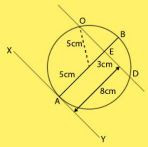As given the question,
AO = OC = 5cm
AM = 8CM
⇒ AM = OM + AO
⇒ OM = AM - AO
Putting these values in the equation,
OM = (8-5)
= 3CM
OM is perpendicular to the chord CD.
In ∆OCM < OMC=90°
By Pythagoras theorem,
OC2 = OM2 + MC2
Therefore,
CD = 2 ×CM
= 8 cm

6. In Fig., AT is a tangent to the circle with centre O such that OT = 4 cm
and ∠OTA 30° . Then AT is equal to
(A) 4 cm
(B) 2 cm
(C) 2√3 cm
(D) 4√3 cm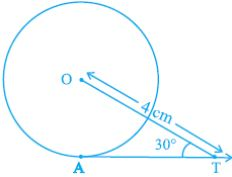Solution
(C)
Join OA
We know that, the tangent at any point of a circle is perpendicular to the radius through the
cos 30° = OT/AT
⇒ √3/2 = AT/4
⇒ AT = 2√3 cm

7. In Fig., if O is the centre of a circle, PQ is a chord and the tangent PR at P makes an angle of 50° with PQ, then ∠POQ is equal to
(A) 100°
(B) 80°
(C) 90°
(D) 75°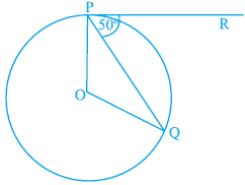Solution
(A)
Given,
∠QPR = 50°
We know that, the tangent at any point of a circle is perpendicular to the radius through the
point of contact.
∠OPR = 90°
⇒ ∠OPQ + ∠QPR = 90°
⇒ ∠OPQ = 90° – 50°
= 40°  [as, ∠QPR = 50°]
Now,
OP = OQ = radius of circle
∠OQP = ∠OPQ
= 40°
[Angles opposite to equal sides are equal]
In ∆OPQ,
∠O + ∠OPQ + ∠Q = 180° [Angle sum property]
⇒ ∠POQ = 180° – (40 + 40°)
= 180° – 80°
[ ∠OPQ = 40° = ∠Q]
⇒ ∠POQ = 100°

8. In Fig., if PA and PB are tangents to the circle with centre O such that ∠APB 50° , then ∠OAB is equal to
(A) 25°
(B) 30°
(C) 40°
(D) 50°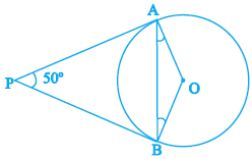Solution
(A)
Given,
PA and PB are tangent lines.
PA = PB  [as, Length of tangents drawn from an external point to a circle is equal]
Let,
∠PBA = ∠PAB = θ
In ∆PAB,
∠P + ∠A + ∠B = 180°  [Angle sum property]
⇒ 50° + θ + θ= 180°
⇒ 2 θ = 180° – 50° = 130°
⇒ θ = 65°
Also,
OA ⊥ PA
[as, tangent at any point of a circle is perpendicular to the radius through the point of contact]
So,
∠PAO = 90°
⇒ ∠PAB + ∠BAO = 90°
⇒ 65° + ∠BAO = 90°
⇒ ∠BAO = 90° – 65° = 25°
⇒ ∠OAB = 25°

9. If two tangents inclined at an angle 60° are drawn to a circle of radius 3 cm, then length of each tangent is equal to
(A) 3√3/2  cm
(B) 6 cm
(C) 3 cm
(D) 3√3 cm
Solution
(D)
Let P be an external point and a pair of tangents is drawn from point P such that the angle between two tangents is 60°.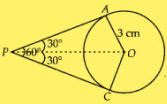Join OA and OP.
Also,
OP is a bisector line of ∠APC.
∠APO = ∠CPO = 30°
And,
OA ⊥ AP
[Tangent at any point of a circle is perpendicular to the radius through the point of contact.]
∠OAP = 90°
In right angled ∆OAP,
tan 30° = OA/AP
⇒ 1/√3 = 3/AP
⇒ AP = 3√3 cm
So, the length of each tangent is 3√3 cm.

10. In Fig., if PQR is the tangent to a circle at Q whose centre is O, AB is a chord parallel to PR and ∠BQR = 70° , then ∠AQB is equal to
(A) 20°
(B) 40°
(C) 35°
(D) 45°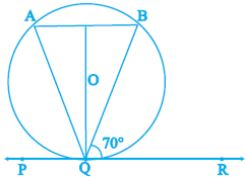Solution
(B)
Given,
AB || PR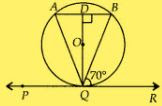Therefore,
∠ABQ = ∠BQR = 70°  [Alternate angles]
Also,
QD is perpendicular to AB and QD bisects AB.
In ∆QDA and ∆QDB,
∠QDA = ∠QDB  [90° each]